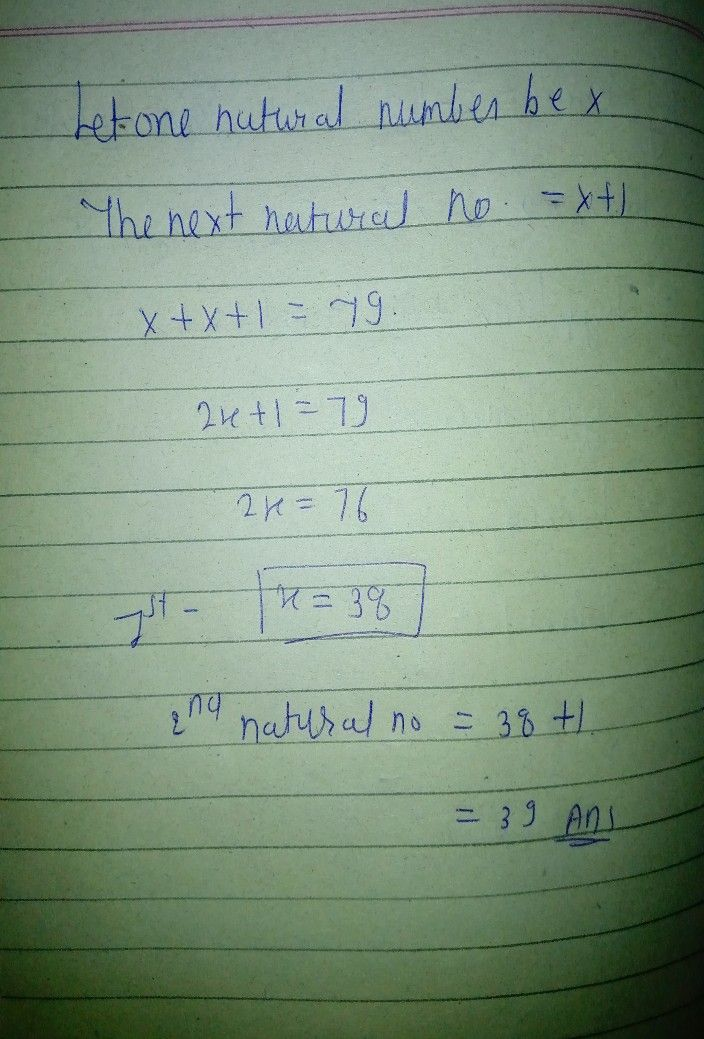Symbol
Problem$7$ The sum of $1$ two consecutive natural numbers is $79$ Find the two numbers. $1$ $Hint$ If $x$ $1$ $1$ $0$ one of the numbers, then the consecutive number $1sx+1$